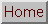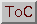## ``` void KLTTrackFeatures( KLT_TrackingContext tc, KLT_PixelType *img1, KLT_PixelType *img2, int ncols, int nrows, KLT_FeatureList fl); ```

KLTTrackFeatures() takes two images pointed to by `img1` and `img2`. (NOTE: `KLT_PixelType` is, unless modified by the user, an `unsigned char`.) If `tc->sequentialMode` is `TRUE`, and KLTTrackFeatures() has been previously called, then `img1` is ignored and the first image is taken instead from `tc->pyramid_last`, `tc->pyramid_last_gradx`, and `tc->pyramid_last_grady`. In either case, the resulting images are smoothed by convolving with a Gaussian of sigma = ```tc->smooth_sigma_fact * max(tc->window_width, tc->window_height)```. Then a multi-resolution image pyramid is created with `tc->nPyramidLevels` levels and `tc->subsampling` pixels subsampled between each level; smoothing before sampling is accomplished with sigma = `tc->subsampling * tc->pyramid_sigma_fact`. Gradients are computed at each level of the pyramid by convolving with the derivative of a Gaussian of sigma = `tc->grad_sigma`.

In the feature list, each feature that is not lost (i.e., whose value is nonnegative) is tracked beginning with the coarsest resolution and ending with the finest resolution, with each resolution providing the starting point for the subsequent resolution. At each resolution, tracking is accomplished by a Newton-Raphson iterative minimization between the intensities of the two windows, one window in each image. There are five conditions that cause the iterations to stop (only in the first case is the tracker successful):

1. the feature moves by no more than `tc->min_displacement`
2. the determinant of the 2-by-2 gradient matrix is less than `tc->min_determinant`
3. the number of iterations exceeds `tc->max_iterations`
4. the feature is out of bounds (i.e., it is within `tc->borderx` or `tc->bordery` of the border of the image)
5. the residue is too large (i.e., the average intensity difference between pixels in the two windows is greater than `tc->max_residue`)
The value of the feature in these cases becomes `KLT_TRACKED`, `KLT_SMALL_DET`, `KLT_MAX_ITERATIONS`, `KLT_OOB`, and `KLT_LARGE_RESIDUE`, respectively, which are in turn equal to 0, -2, -3, -4, and -5. (See the reference page of `KLT_Feature` to see what a value of -1 means.)

If `tc->writeInternalImages` is `TRUE`, then the smoothed image and the image derivatives at each level of the pyramid are written to `"kltimg_tf_[I][n].pgm"`, `"kltimg_tf_[I][n]_gx.pgm"`, and `"kltimg_tf_[I][n]_gy.pgm"`, where `[I] = i,j` denotes either the first image or the second image, and `[n] = 0,1,2,...` denotes the level of the pyramid.

After all the features are tracked, if `tc->sequentialMode` is `TRUE`, then the second image and its derivatives are stored in `tc->pyramid_last`, `tc->pyramid_last_gradx`, and `tc->pyramid_last_grady`.